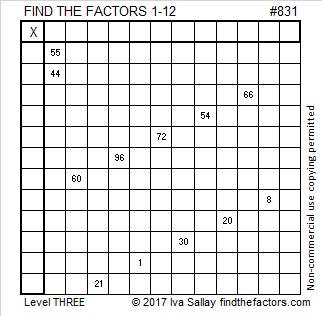# 831 and Level 3Print the puzzles or type the solution on this excel file: 12 factors 829-834

Before I write a blog post, I look to see how the post number is expressed in different bases. Today I noticed that 831 is 30333 in BASE 4. I was intrigued by all those 3’s because I knew that 831 = 3 × 277. It seems logical that 277 would be 10111 in BASE 4, it turns out that it is! I looked at 831 in all the bases up to BASE 36. Did any others have only multiples of 3 as its digits? Yes, a few did, so I’ve made a chart of 277 and 831 in those five bases to make comparing them easy. I also used only base 10 numbers and not letters of the alphabet to represent the digits in the other bases. As you look at this chart, remember 3 × 277 = 831.Why are those the ONLY bases for which 3 times the digits of 277 equals the digits for 831? Because in every other base, at least one of the digits times 3 will be greater than or equal to the base and some complicated carrying will have to take place to determine the digits for 831 in that base.

For example, 277 is palindrome 1 11 1 in BASE 12. Obviously 3 times 1 11 1 is 3 33 3. Since 33 is bigger than 12, we somehow end up with non-palindrome 5 9 3 in BASE 12 for 831. This is how that somehow happened: 33÷12 = 2R9. The 9 becomes the middle digit while the 2 is added to the original 3 to make the first digit, 5.

Here’s a little more about the number 831:

Because 277 is one of its factors, 831 is the hypotenuse of a Pythagorean triple: 345-756-831, which is 3 times primitive (115-252-277).

• 831 is a composite number.
• Prime factorization: 831 = 3 × 277
• The exponents in the prime factorization are 1 and 1. Adding one to each and multiplying we get (1 + 1)(1 + 1) = 2 × 2 = 4. Therefore 831 has exactly 4 factors.
• Factors of 831: 1, 3, 277, 831
• Factor pairs: 831 = 1 × 831 or 3 × 277
• 831 has no square factors that allow its square root to be simplified. √831 ≈ 8270706